# IB Chemistry ATOMIC THEORY Atomic Structure Atomic Structure

• Slides: 63IB Chemistry ATOMIC THEORY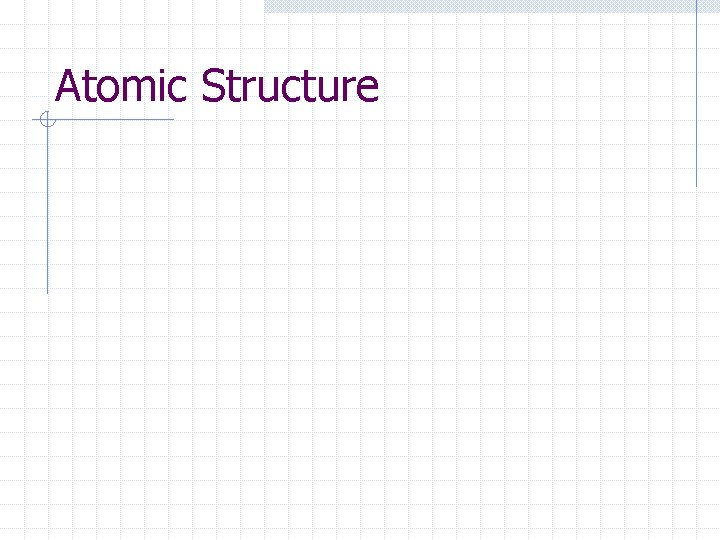Atomic Structure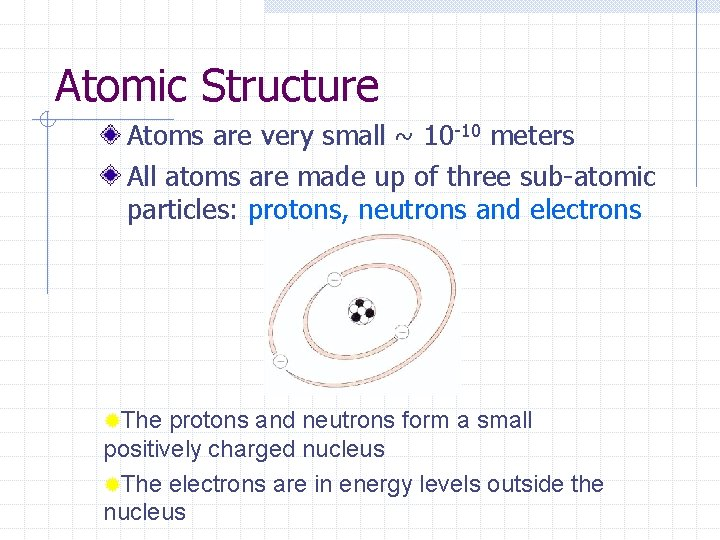Atomic Structure Atoms are very small ~ 10 -10 meters All atoms are made up of three sub-atomic particles: protons, neutrons and electrons ®The protons and neutrons form a small positively charged nucleus ®The electrons are in energy levels outside the nucleusAtomic Structure The actual values of the masses and charges of the sub-atomic particles are shown below: ®A meaningful way to consider the masses of the subatomic particles is to use relative masses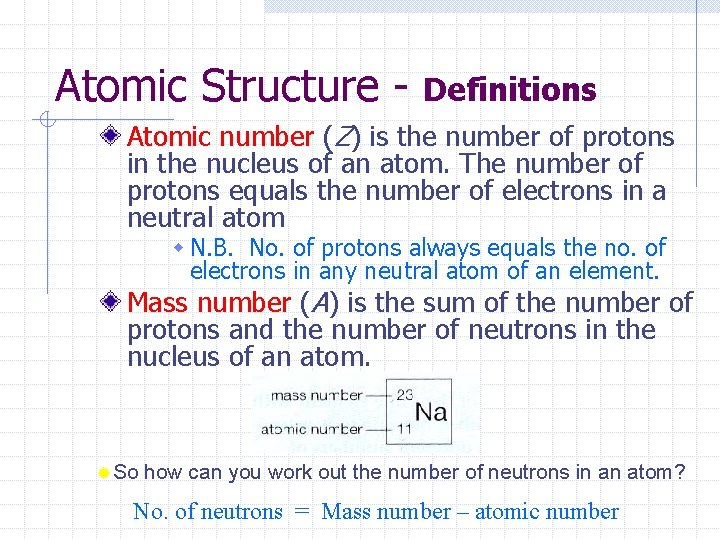Atomic Structure - Definitions Atomic number (Z) is the number of protons in the nucleus of an atom. The number of protons equals the number of electrons in a neutral atom w N. B. No. of protons always equals the no. of electrons in any neutral atom of an element. Mass number (A) is the sum of the number of protons and the number of neutrons in the nucleus of an atom. ®So how can you work out the number of neutrons in an atom? No. of neutrons = Mass number – atomic numberAtomic Structure - Example ®So how can you work out the number of neutrons in an atom? ®Example No. of neutrons = Mass number – atomic number No. of neutron = Mass No. – Atomic No. = 23 – 11 = 12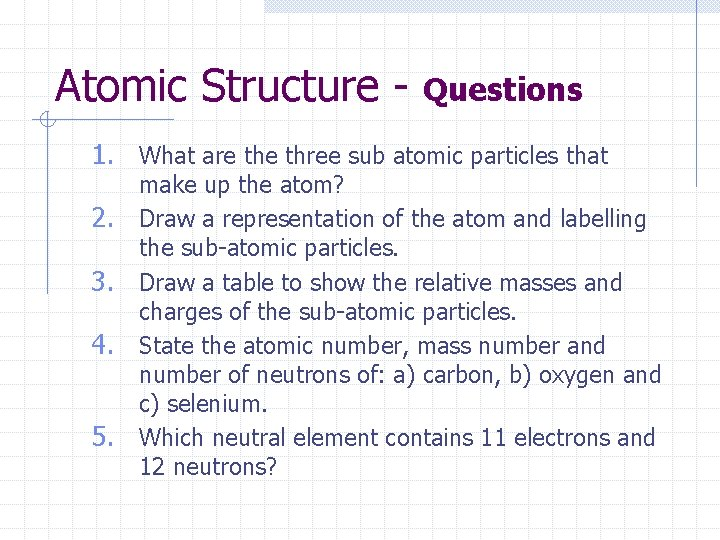Atomic Structure - Questions 1. What are three sub atomic particles that 2. 3. 4. 5. make up the atom? Draw a representation of the atom and labelling the sub-atomic particles. Draw a table to show the relative masses and charges of the sub-atomic particles. State the atomic number, mass number and number of neutrons of: a) carbon, b) oxygen and c) selenium. Which neutral element contains 11 electrons and 12 neutrons?Atomic Structure - Questions 5. Copy and complete the following table: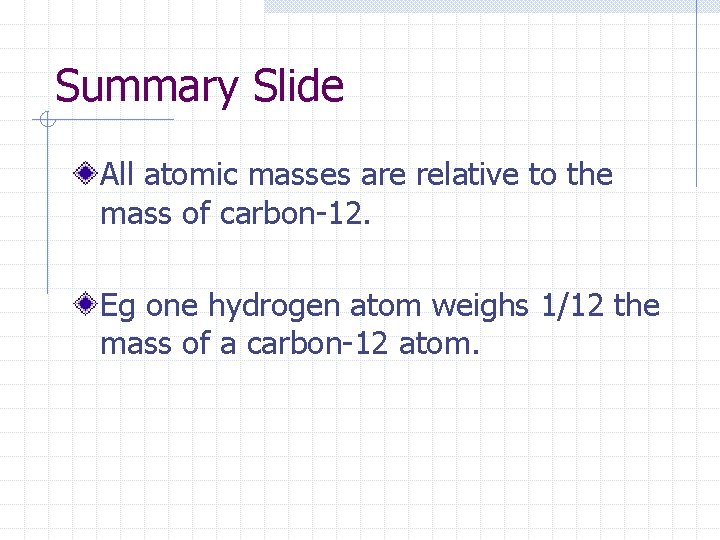Summary Slide All atomic masses are relative to the mass of carbon-12. Eg one hydrogen atom weighs 1/12 the mass of a carbon-12 atom.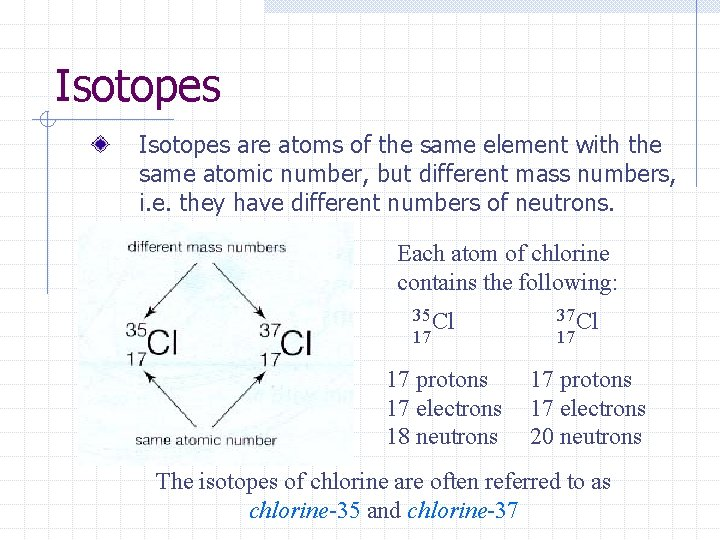Isotopes are atoms of the same element with the same atomic number, but different mass numbers, i. e. they have different numbers of neutrons. Each atom of chlorine contains the following: 35 Cl 17 17 protons 17 electrons 18 neutrons 37 Cl 17 17 protons 17 electrons 20 neutrons The isotopes of chlorine are often referred to as chlorine-35 and chlorine-37Isotopes of an element have the same chemical properties because they have the same number of electrons. When a chemical reaction takes place, it is the electrons that are involved in the reactions. However isotopes of an element have the slightly different physical properties because they have different numbers of neutrons, hence different masses. The isotopes of an element with fewer neutrons will have: w w Lower masses Lower densities • • faster rate of diffusion lower melting and boiling points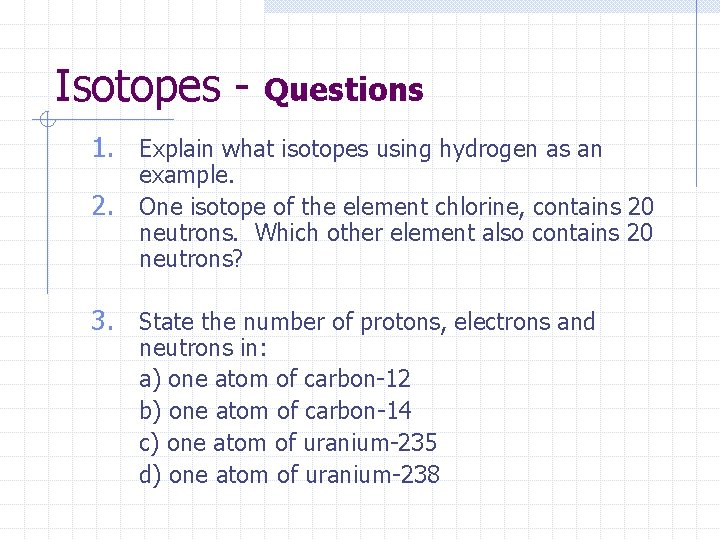Isotopes - Questions 1. Explain what isotopes using hydrogen as an 2. example. One isotope of the element chlorine, contains 20 neutrons. Which other element also contains 20 neutrons? 3. State the number of protons, electrons and neutrons in: a) one atom of carbon-12 b) one atom of carbon-14 c) one atom of uranium-235 d) one atom of uranium-238Mass Spectrometer The mass spectrometer is an instrument used: w To measure the relative masses of isotopes w To find the relative abundance of the isotopes in a sample of an element When charged particles pass through a magnetic field, the particles are deflected by the magnetic field, and the amount of deflection depends upon the mass/charge ratio of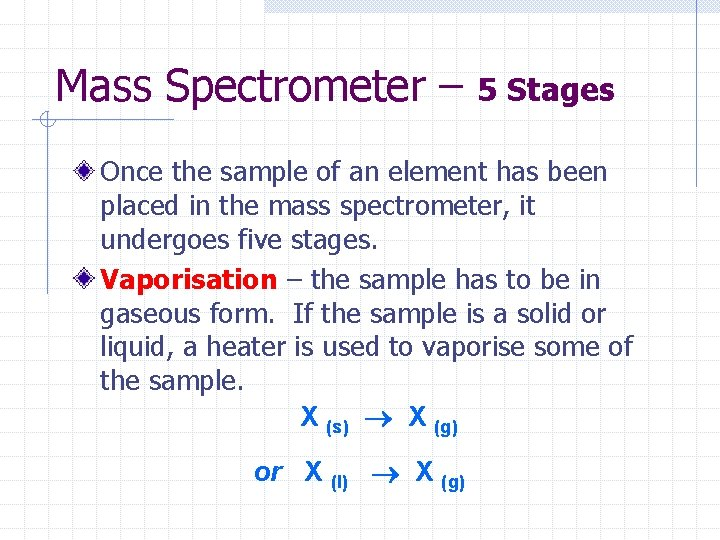Mass Spectrometer – 5 Stages Once the sample of an element has been placed in the mass spectrometer, it undergoes five stages. Vaporisation – the sample has to be in gaseous form. If the sample is a solid or liquid, a heater is used to vaporise some of the sample. X (s) X (g) or X (l) X (g)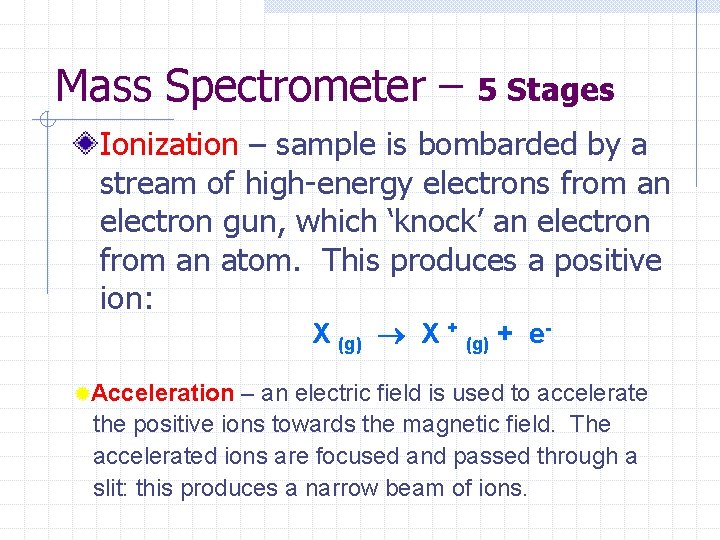Mass Spectrometer – 5 Stages Ionization – sample is bombarded by a stream of high-energy electrons from an electron gun, which ‘knock’ an electron from an atom. This produces a positive ion: X (g) X + (g) + e- ®Acceleration – an electric field is used to accelerate the positive ions towards the magnetic field. The accelerated ions are focused and passed through a slit: this produces a narrow beam of ions.Mass Spectrometer – 5 Stages Deflection – The accelerated ions are deflected into the magnetic field. The amount of deflection is greater when: • the mass of the positive ion is less • the charge on the positive ion is greater • the velocity of the positive ion is less • the strength of the magnetic fieldMass Spectrometer If all the ions are travelling at the same velocity and carry the same charge, the amount of deflection in a given magnetic field depends upon the mass of the ion. For a given magnetic field, only ions with a particular relative mass (m) to charge (z) ration – the m/z value – are deflected sufficiently to reach the detector.Mass Spectrometer Detection – ions that reach the detector cause electrons to be released in an ioncurrent detector The number of electrons released, hence the current produced is proportional to the number of ions striking the detector. The detector is linked to an amplifier and then to a recorder: this converts the current into a peak which is shown in the mass spectrum.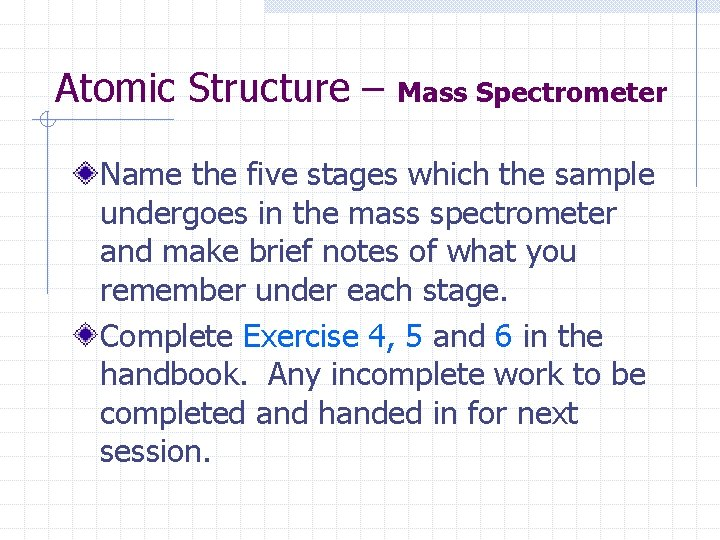Atomic Structure – Mass Spectrometer Name the five stages which the sample undergoes in the mass spectrometer and make brief notes of what you remember under each stage. Complete Exercise 4, 5 and 6 in the handbook. Any incomplete work to be completed and handed in for next session.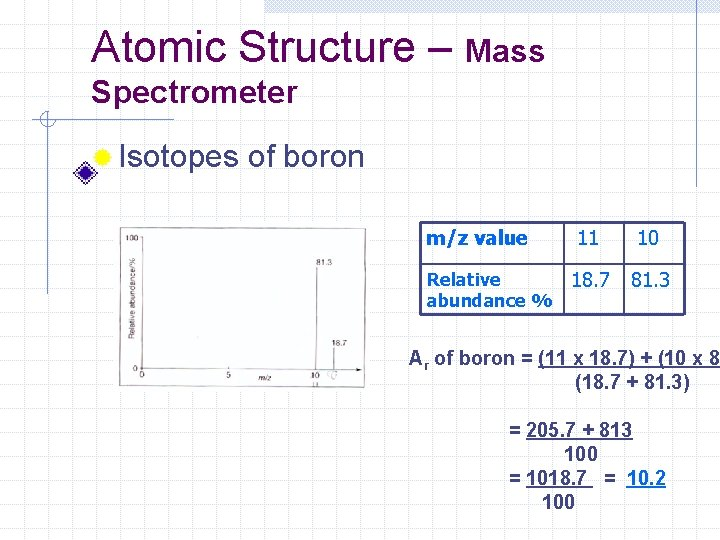Atomic Structure – Mass Spectrometer ® Isotopes of boron m/z value Relative abundance % 11 10 18. 7 81. 3 Ar of boron = (11 x 18. 7) + (10 x 81 (18. 7 + 81. 3) = 205. 7 + 813 100 = 1018. 7 = 10. 2 100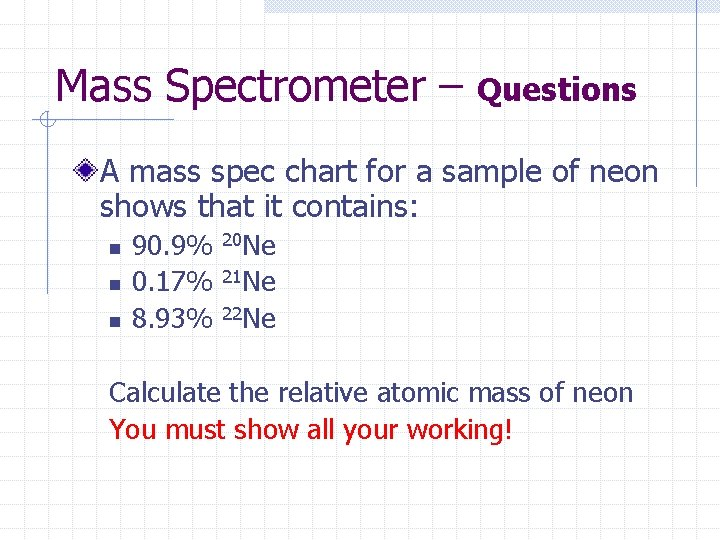Mass Spectrometer – Questions A mass spec chart for a sample of neon shows that it contains: n n n 90. 9% 0. 17% 8. 93% 20 Ne 21 Ne 22 Ne Calculate the relative atomic mass of neon You must show all your working!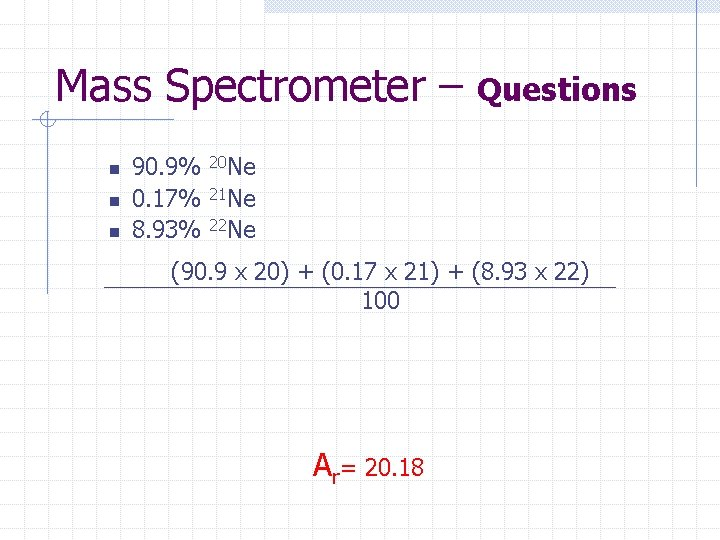Mass Spectrometer – n n n 90. 9% 0. 17% 8. 93% Questions 20 Ne 21 Ne 22 Ne (90. 9 x 20) + (0. 17 x 21) + (8. 93 x 22) 100 Ar= 20. 18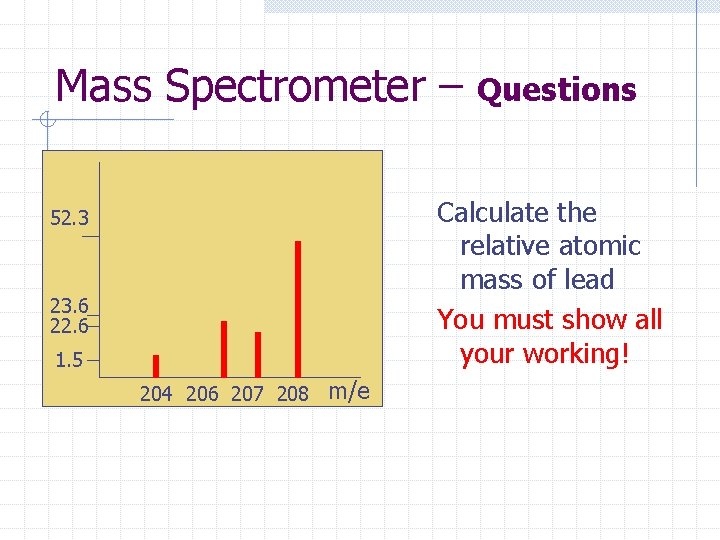Mass Spectrometer – Questions Calculate the relative atomic mass of lead You must show all your working! 52. 3 23. 6 22. 6 1. 5 204 206 207 208 m/eMass Spectrometer – n n Questions 1. 5% 204 Pb 23. 6% 206 Pb 22. 6% 207 Pb 52. 3% 208 Pb (1. 5 x 204) + (23. 6 x 206) + (22. 6 x 207)+(52. 3 x 208) 100 306 + 4861. 6 + 4678. 2 + 10878. 4 20724. 2 100 Ar= 207. 24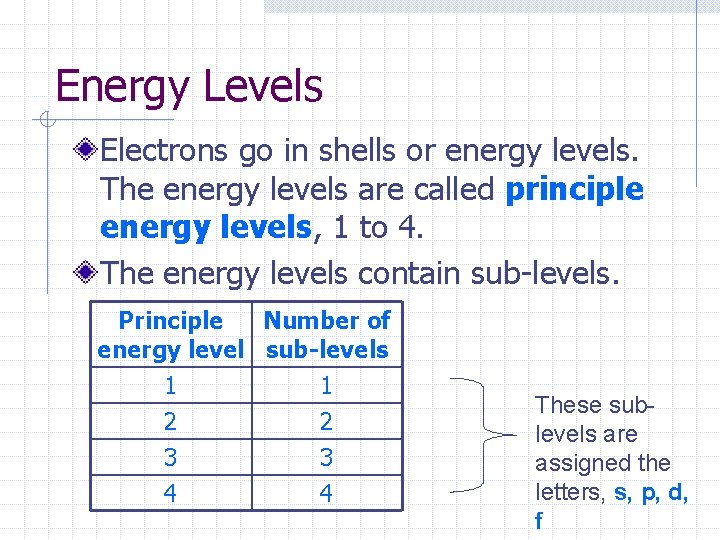Energy Levels Electrons go in shells or energy levels. The energy levels are called principle energy levels, 1 to 4. The energy levels contain sub-levels. Principle Number of energy level sub-levels 1 2 3 4 These sublevels are assigned the letters, s, p, d, f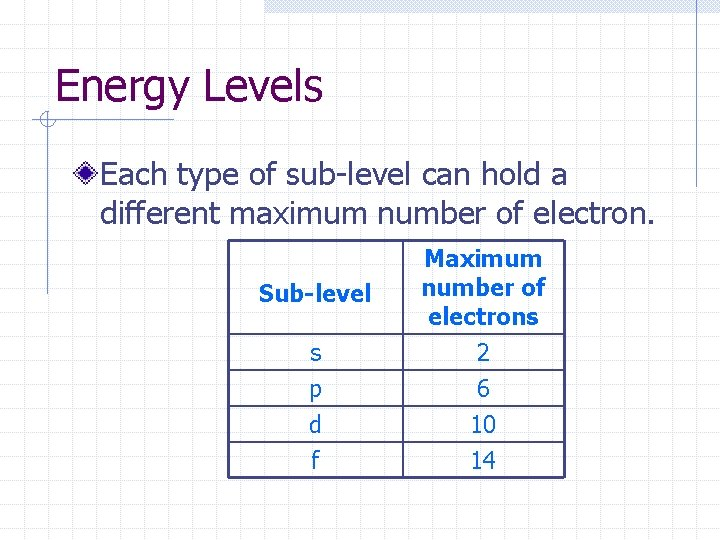Energy Levels Each type of sub-level can hold a different maximum number of electron. Sub-level Maximum number of electrons s p d f 2 6 10 14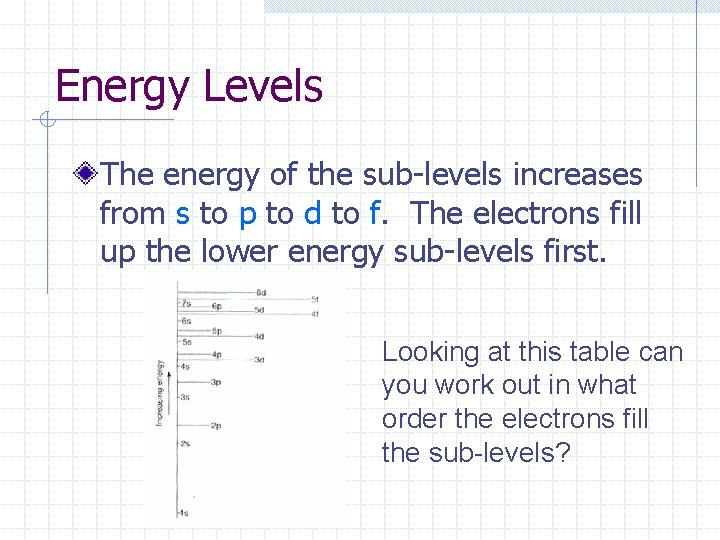Energy Levels The energy of the sub-levels increases from s to p to d to f. The electrons fill up the lower energy sub-levels first. Looking at this table can you work out in what order the electrons fill the sub-levels?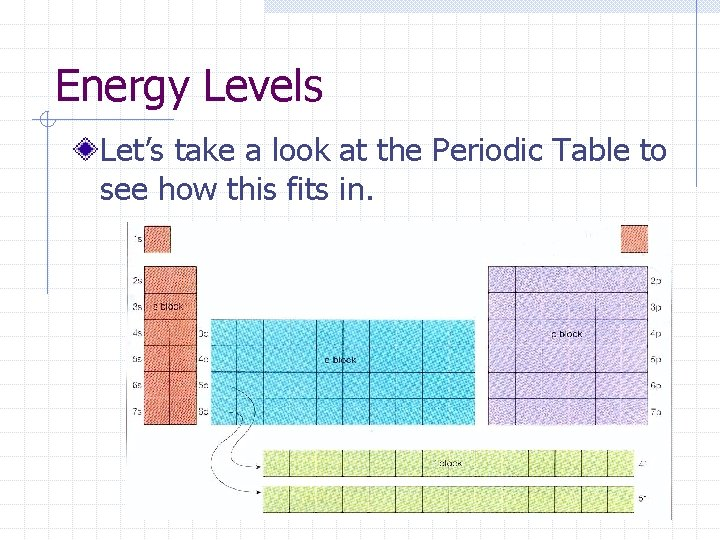Energy Levels Let’s take a look at the Periodic Table to see how this fits in.Electronic Structure So how do you write it? 1 s 2 Energy level Sub-level Example For magnesium: 1 s 2, 2 p 6, 3 s 2 Number of electrons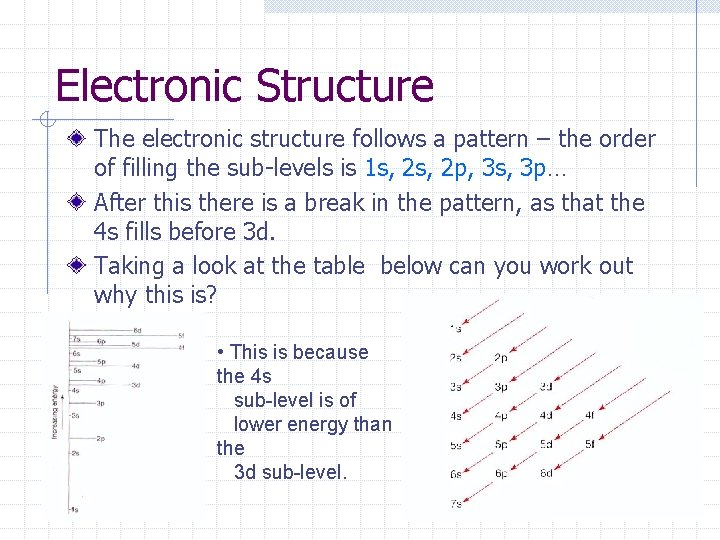Electronic Structure The electronic structure follows a pattern – the order of filling the sub-levels is 1 s, 2 p, 3 s, 3 p… After this there is a break in the pattern, as that the 4 s fills before 3 d. Taking a look at the table below can you work out why this is? • This is because the 4 s sub-level is of lower energy than the 3 d sub-level.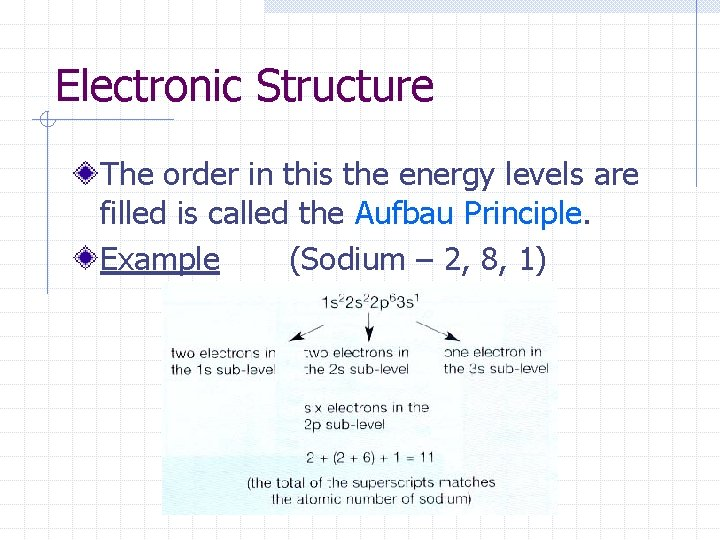Electronic Structure The order in this the energy levels are filled is called the Aufbau Principle. Example (Sodium – 2, 8, 1)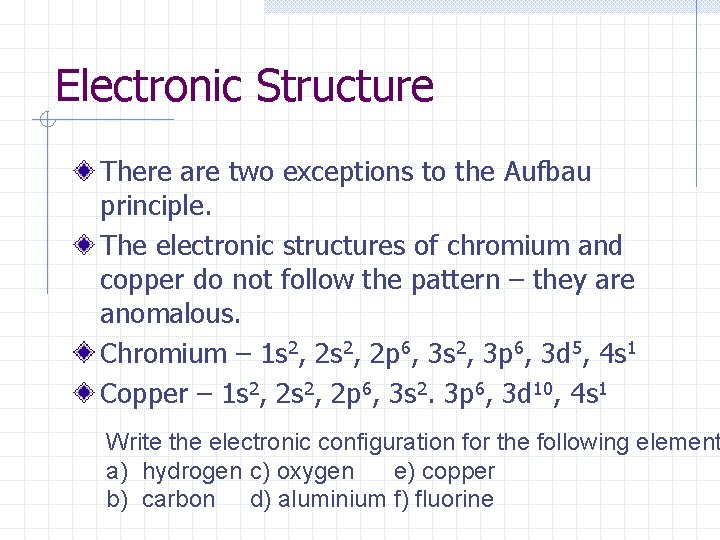Electronic Structure There are two exceptions to the Aufbau principle. The electronic structures of chromium and copper do not follow the pattern – they are anomalous. Chromium – 1 s 2, 2 p 6, 3 s 2, 3 p 6, 3 d 5, 4 s 1 Copper – 1 s 2, 2 p 6, 3 s 2. 3 p 6, 3 d 10, 4 s 1 Write the electronic configuration for the following element a) hydrogen c) oxygen e) copper b) carbon d) aluminium f) fluorine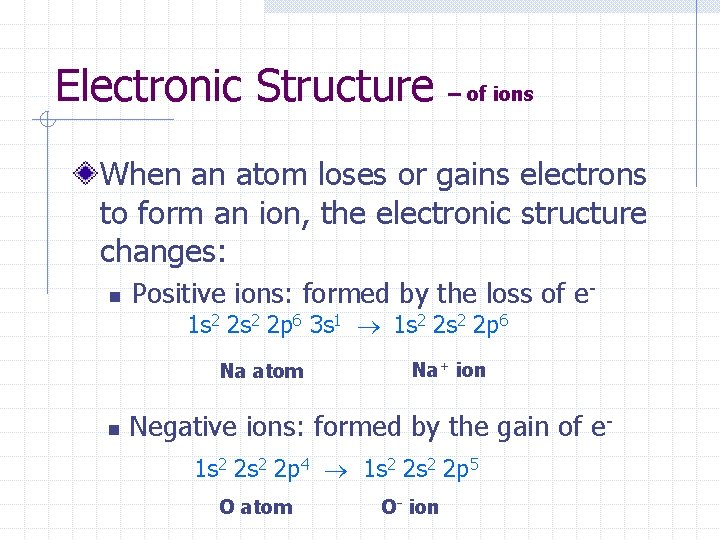Electronic Structure – of ions When an atom loses or gains electrons to form an ion, the electronic structure changes: n Positive ions: formed by the loss of e 1 s 2 2 p 6 3 s 1 1 s 2 2 p 6 Na atom n Na+ ion Negative ions: formed by the gain of e 1 s 2 2 p 4 1 s 2 2 p 5 O atom O- ionElectronic Structure – of transition metals With the transition metals it is the 4 s electrons that are lost first when they form ions: n Titanium (Ti) - loss of 2 e- 1 s 2 2 p 6 3 s 2 3 p 6 3 d 2 4 s 2 1 s 2 2 p 6 3 s 2 3 p 6 3 d 2 Ti atom n Ti 2+ ion Chromium (Cr) - loss of 3 e- 1 s 2 2 p 6 3 s 2 3 p 6 3 d 5 4 s 1 1 s 2 2 p 6 3 s 2 3 p 6 3 d 3 Cr atom Cr 3+ ionElectronic Structure - Questions Give the full electronic structure of the following positve ions: a) Mg 2+ b) Ca 2+ c) Al 3+ Give the full electronic structure of the negative ions: a) Clb) Brc) P 3 -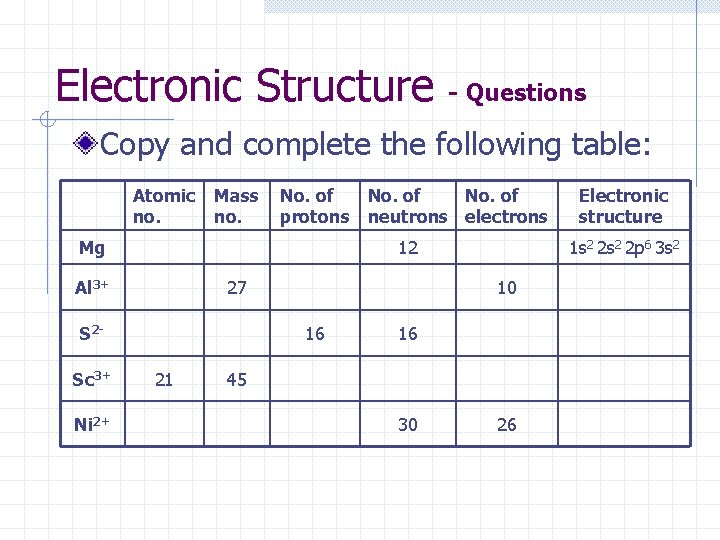Electronic Structure - Questions Copy and complete the following table: Atomic no. Mass no. No. of protons neutrons electrons Mg 12 Al 3+ 27 S 2 Sc 3+ Ni 2+ 21 1 s 2 2 p 6 3 s 2 10 16 16 45 30 Electronic structure 26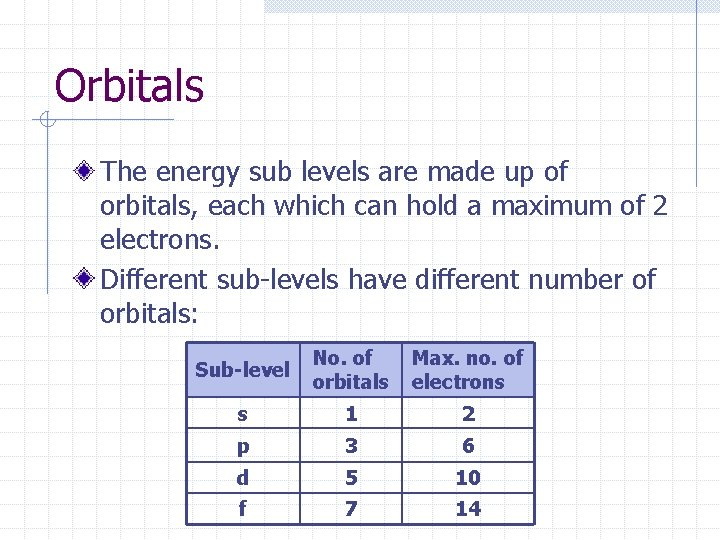Orbitals The energy sub levels are made up of orbitals, each which can hold a maximum of 2 electrons. Different sub-levels have different number of orbitals: Sub-level No. of orbitals Max. no. of electrons s 1 2 p 3 6 d 5 10 f 7 14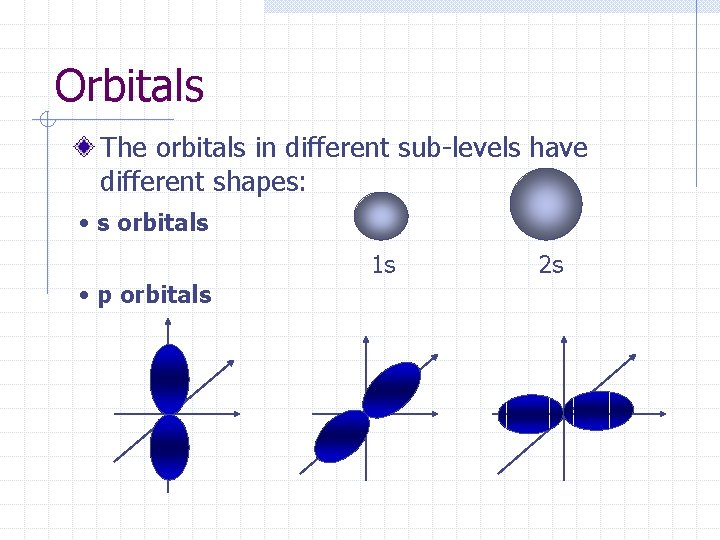Orbitals The orbitals in different sub-levels have different shapes: • s orbitals 1 s • p orbitals 2 sOrbitals Within a sub-level, the electrons occupy orbitals as unpaired electrons rather than paired electrons. (This is known as Hund’s Rule). We use boxes to represent orbitals: 2 p 1 s 2 s Electronic structure of carbon, 1 s 2, 2 p 2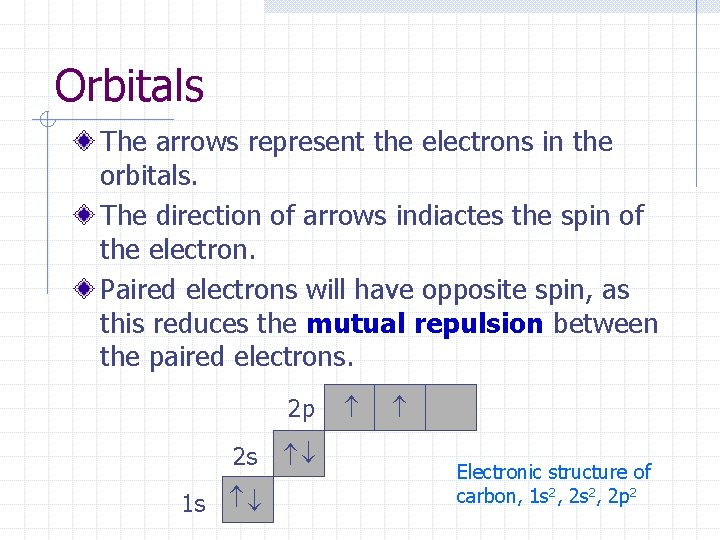Orbitals The arrows represent the electrons in the orbitals. The direction of arrows indiactes the spin of the electron. Paired electrons will have opposite spin, as this reduces the mutual repulsion between the paired electrons. 2 p 1 s 2 s Electronic structure of carbon, 1 s 2, 2 p 2Orbitals Using boxes to represent orbitals, give the full electronic structure of the following atoms: a) lithium d) nitrogen b) fluorine e) oxygen 2 p 2 s 1 s c) potassium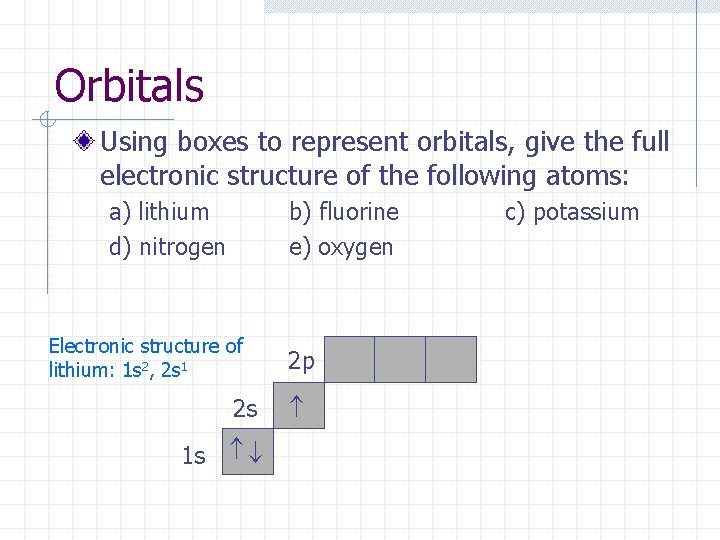Orbitals Using boxes to represent orbitals, give the full electronic structure of the following atoms: a) lithium d) nitrogen b) fluorine e) oxygen Electronic structure of lithium: 1 s 2, 2 s 1 2 p 2 s 1 s c) potassiumOrbitals Using boxes to represent orbitals, give the full electronic structure of the following atoms: b) fluorine e) oxygen 2 p 1 s 2 s Electronic structure of fluorine: 1 s 2, 2 p 5 c) potassium a) lithium d) nitrogenOrbitals Using boxes to represent orbitals, give the full electronic structure of the following atoms: a) lithium d) nitrogen b) fluorine e) oxygen 4 s Electronic structure of potassium: 1 s 2, 2 p 6, 3 s 2, 3 p 6, 4 s 1 3 p 3 s 2 p 2 s 1 s c) potassiumOrbitals Using boxes to represent orbitals, give the full electronic structure of the following atoms: a) lithium d) nitrogen b) fluorine e) oxygen Electronic structure of nitrogen: 1 s 2, 2 p 3 2 p 1 s 2 s c) potassiumOrbitals Using boxes to represent orbitals, give the full electronic structure of the following atoms: a) lithium d) nitrogen b) fluorine e) oxygen Electronic structure of oxygen: 1 s 2, 2 p 4 2 p 1 s 2 s c) potassium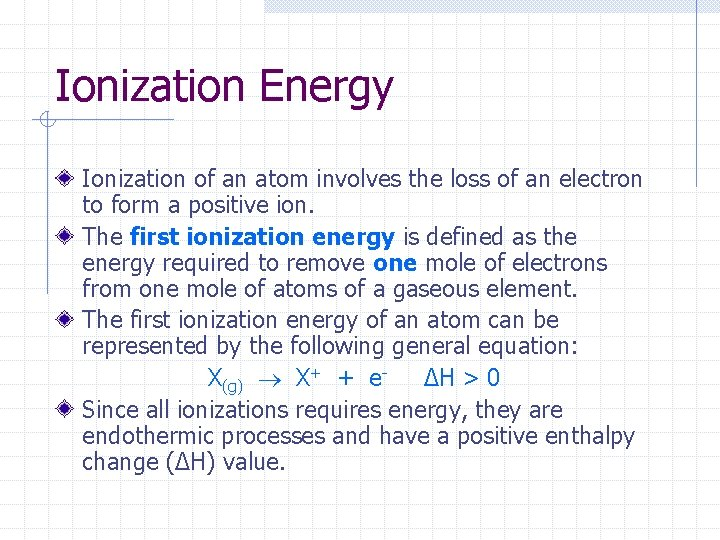Ionization Energy Ionization of an atom involves the loss of an electron to form a positive ion. The first ionization energy is defined as the energy required to remove one mole of electrons from one mole of atoms of a gaseous element. The first ionization energy of an atom can be represented by the following general equation: X(g) X+ + eΔH > 0 Since all ionizations requires energy, they are endothermic processes and have a positive enthalpy change (ΔH) value.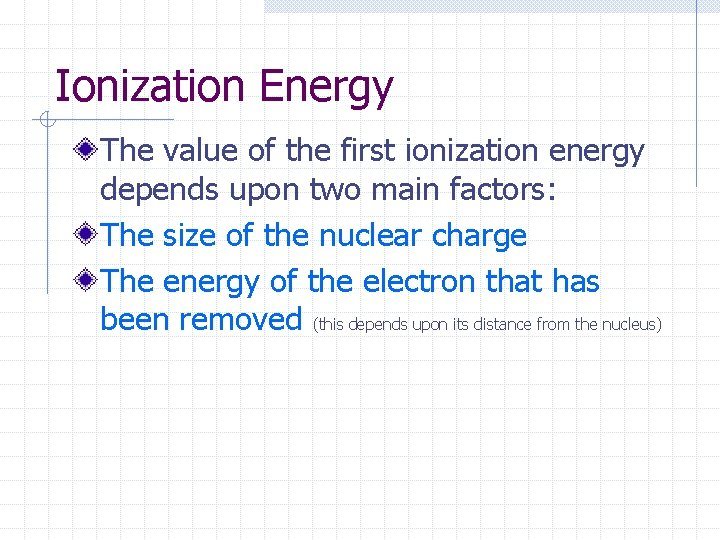Ionization Energy The value of the first ionization energy depends upon two main factors: The size of the nuclear charge The energy of the electron that has been removed (this depends upon its distance from the nucleus)Ionization Energy As the size of the nuclear charge increases the force of the attraction between the negatively charged electrons and the positively charged nucleus increases. Small nuclear charge Small force of attraction Smaller ionization energy + + Large nuclear charge Large force of attraction Greater ionization energyIonization energy As the energy of the electron increases, the electron is farther away from the nucleus. As a result the force of attraction between the nucleus and the electron decreases. Electrons closer to positive nucleus Large force of attraction Greater ionization energy + + Electrons further away from positive nucleus Small force of attraction Smaller ionization energy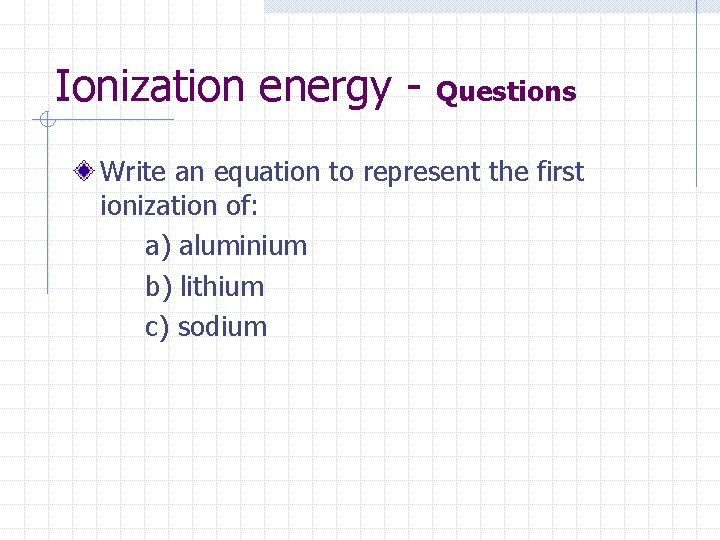Ionization energy - Questions Write an equation to represent the first ionization of: a) aluminium b) lithium c) sodium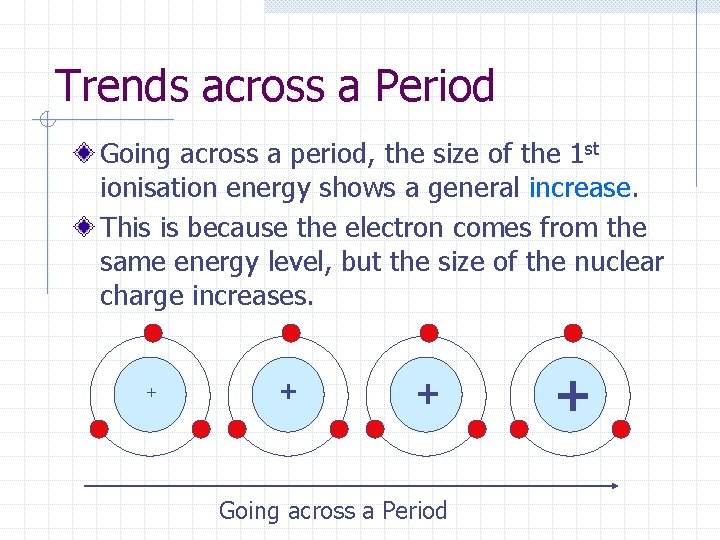Trends across a Period Going across a period, the size of the 1 st ionisation energy shows a general increase. This is because the electron comes from the same energy level, but the size of the nuclear charge increases. + + + Going across a Period +Trends across a Period (2 exceptions) The first ionisation of Al is less than that of Mg, despite the increase in the nuclear charge. The reason for this is that the outer electron removed from Al is in a higher sub-level: the electron removed from Al is a 3 p electron, whereas that removed from Mg is a 3 s. Electronic structure Ionisation energy/k. J mol -1 Na 1 s 2, 2 p 6, 3 s 1 494 Mg 1 s 2, 2 p 6, 3 s 2 736 Al 1 s 2, 2 p 6, 3 s 2, 3 p 1 577 Si 1 s 2, 2 p 6, 3 s 2, 3 p 2 786 P 1 s 2, 2 p 6, 3 s 2, 3 p 3 1060 S 1 s 2, 2 p 6, 3 s 2, 3 p 4 1000 Cl 1 s 2, 2 p 6, 3 s 2, 3 p 5 1260 Ar 1 s 2, 2 p 6, 3 s 2, 3 p 6 1520Trends across a Period (2 exceptions) The first ionisation energy of S is less than that of P, despite the increase in the nuclear charge. In both cases the electron removed is from the 3 p sublevel. However the 3 p electron removed from S is a paired electron, whereas the 3 p electron removed from P is an unpaired electron. When the electrons are paired the extra mutual repulsion results in less energy being required to remove an electron, hence a reduction in the ionisation energy. Sulphur Phosphorus 3 p 3 s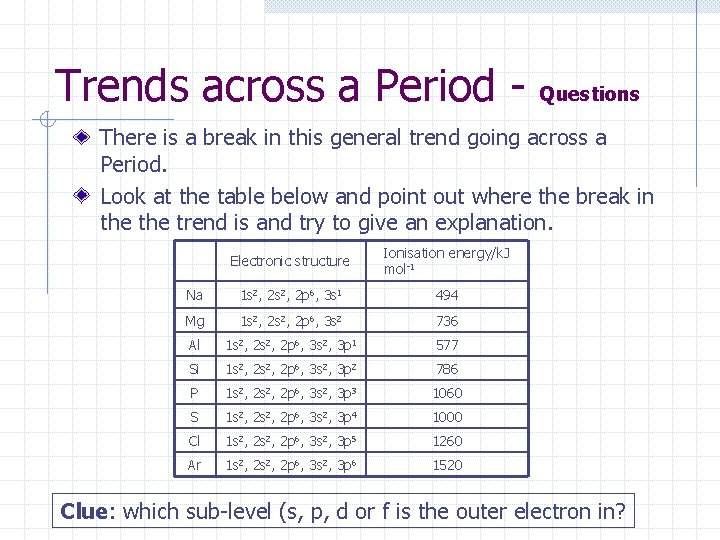Trends across a Period - Questions There is a break in this general trend going across a Period. Look at the table below and point out where the break in the trend is and try to give an explanation. Electronic structure Ionisation energy/k. J mol-1 Na 1 s 2, 2 p 6, 3 s 1 494 Mg 1 s 2, 2 p 6, 3 s 2 736 Al 1 s 2, 2 p 6, 3 s 2, 3 p 1 577 Si 1 s 2, 2 p 6, 3 s 2, 3 p 2 786 P 1 s 2, 2 p 6, 3 s 2, 3 p 3 1060 S 1 s 2, 2 p 6, 3 s 2, 3 p 4 1000 Cl 1 s 2, 2 p 6, 3 s 2, 3 p 5 1260 Ar 1 s 2, 2 p 6, 3 s 2, 3 p 6 1520 Clue: which sub-level (s, p, d or f is the outer electron in?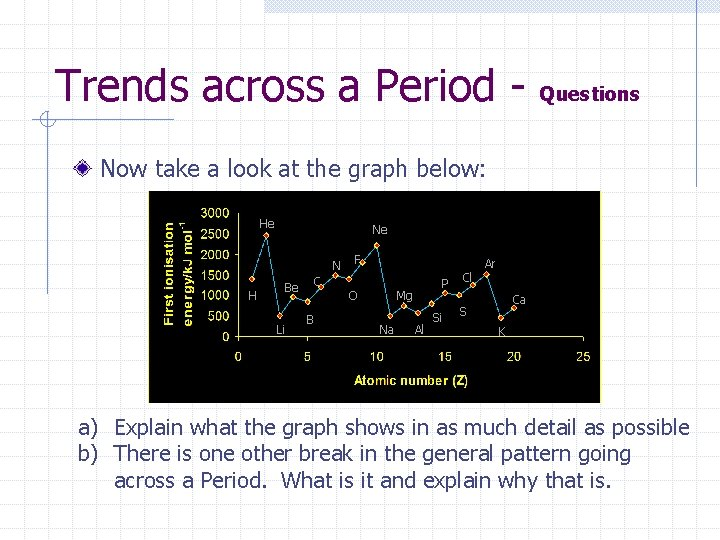Trends across a Period - Questions Now take a look at the graph below: He Ne N H Be Li C B F O P Mg Na Al Si Cl Ar Ca S K a) Explain what the graph shows in as much detail as possible b) There is one other break in the general pattern going across a Period. What is it and explain why that is.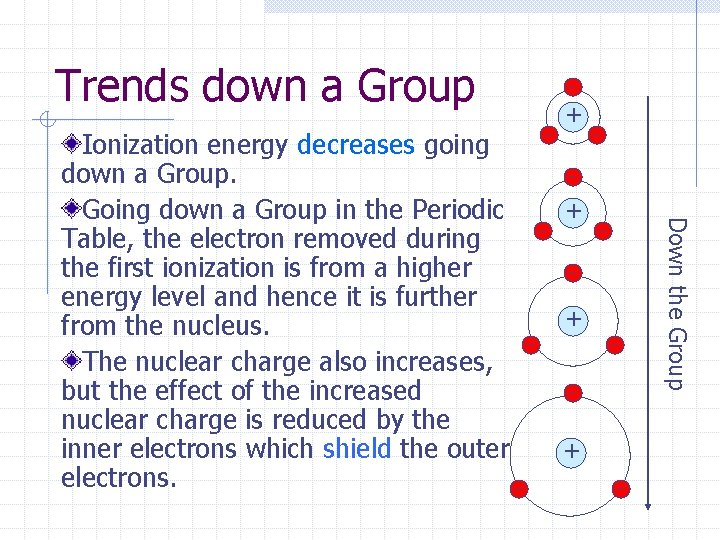Trends down a Group + + + Down the Group Ionization energy decreases going down a Group. Going down a Group in the Periodic Table, the electron removed during the first ionization is from a higher energy level and hence it is further from the nucleus. The nuclear charge also increases, but the effect of the increased nuclear charge is reduced by the inner electrons which shield the outer electrons. +Ionization energy - Questions 1. Explain why sodium has a higher first ionization energy than potassium. 2. Explain why the first ionization energy of boron is less than that of beryllium. 3. Why does helium have the highest first ionisation energy of all the elements? 4. Complete Tasks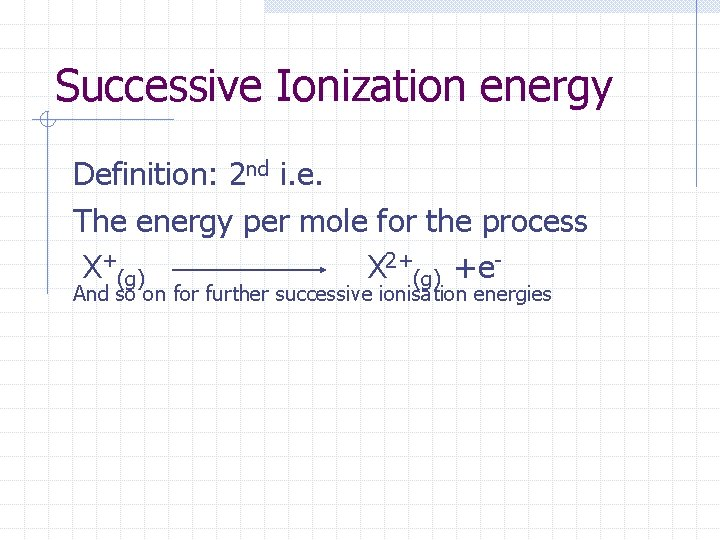Successive Ionization energy Definition: 2 nd i. e. The energy per mole for the process X+(g) X 2+(g) +e. And so on for further successive ionisation energies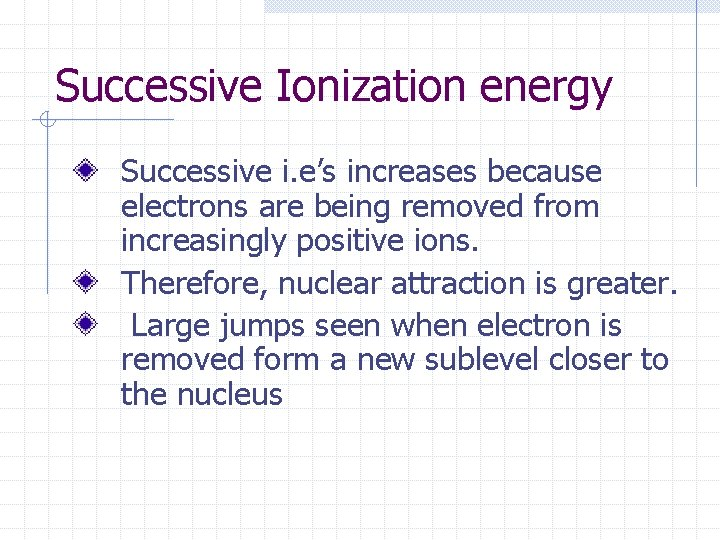Successive Ionization energy Successive i. e’s increases because electrons are being removed from increasingly positive ions. Therefore, nuclear attraction is greater. Large jumps seen when electron is removed form a new sublevel closer to the nucleusSuccessive Ionization energy Large increase between 4 th and 3 rd shells – electron closer to nucleus 2 nd i. e higher than first – electron has greater pull from nucleus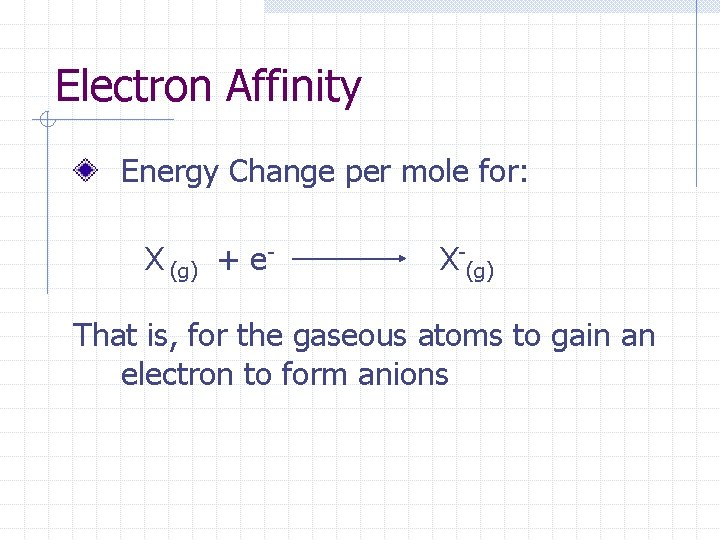Electron Affinity Energy Change per mole for: X (g) + e- X-(g) That is, for the gaseous atoms to gain an electron to form anions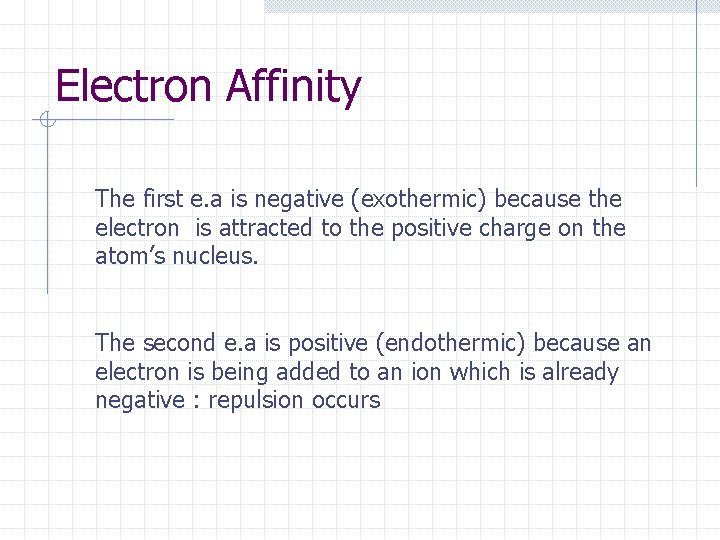Electron Affinity The first e. a is negative (exothermic) because the electron is attracted to the positive charge on the atom’s nucleus. The second e. a is positive (endothermic) because an electron is being added to an ion which is already negative : repulsion occurs